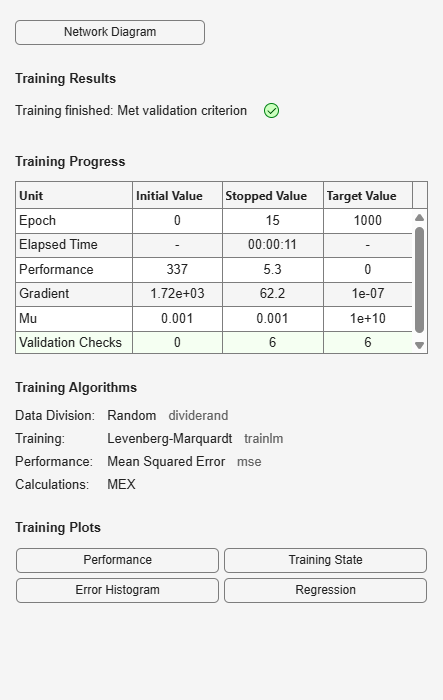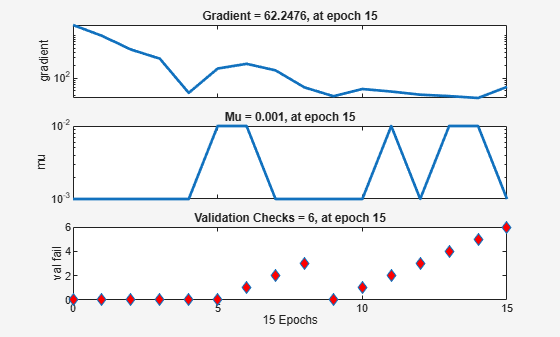# plottrainstate

Plot training state values

## Syntax

```plottrainstate(tr) ```

## Description

`plottrainstate(tr)` plots the training state from a training record `tr` returned by `train`.

## Examples

collapse all

This example shows how to plot training state values using `plottrainstate`.

```[x, t] = bodyfat_dataset; net = feedforwardnet(10); [net, tr] = train(net, x, t);````plottrainstate(tr)`## Version History

Introduced in R2008a